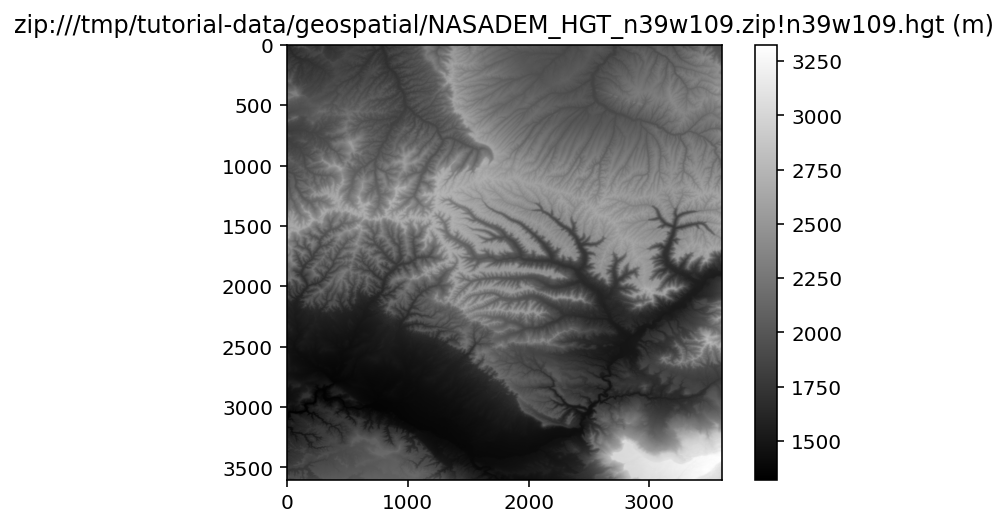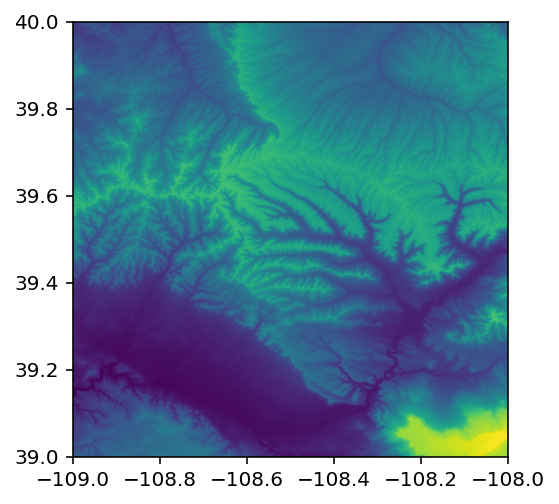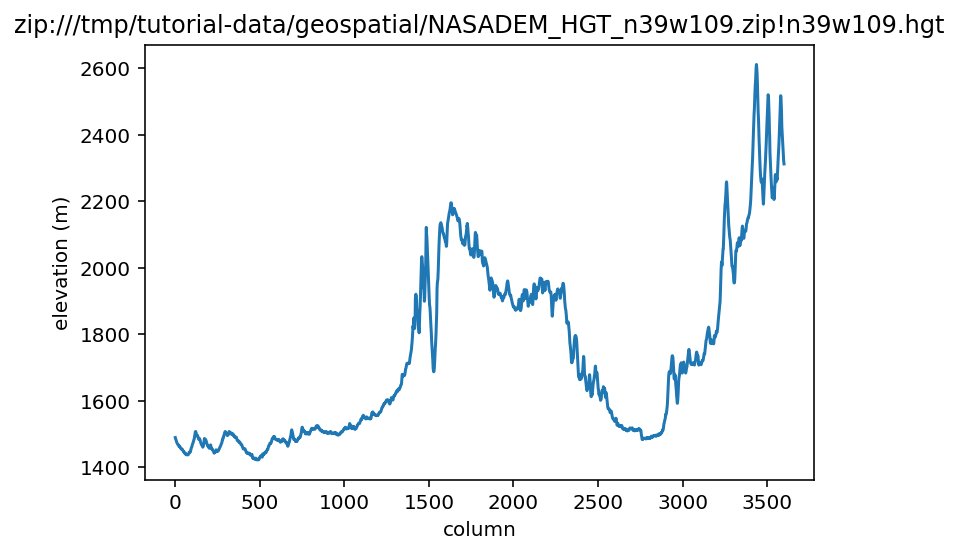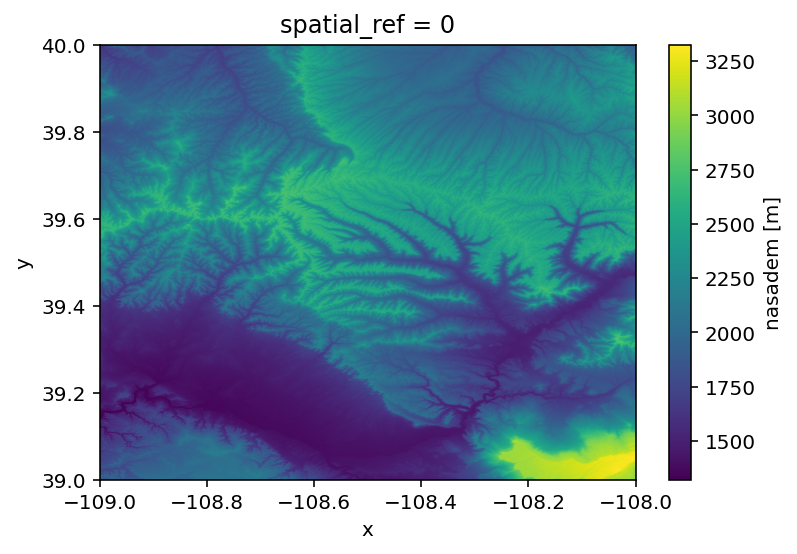# Raster data¶

Learning Objectives

A 30 minute guide to raster data for SnowEX

• find, visualize, interpret raster data formats

• reproject rasters to different coordinate reference systems

• use Python raster libraries rasterio and rioxarray

## Raster Basics¶

Raster data is stored as a grid of values which are rendered on a map as pixels. Each pixel value represents an area on the Earth’s surface. Pixel values can be continuous (elevation) or categorical (land use). This data structure is very common - jpg images on the web, photos from your digital camera. A geospatial raster is only unique in that it is accompanied by metadata that connects the pixel grid to a location on Earth’s surface.

### Coordinate Reference System or “CRS”¶

This specifies the datum, projection, and additional parameters needed to place the raster in geographic space. For a dedicated lesson on CRSs, see: https://datacarpentry.org/organization-geospatial/03-crs/index.html

The natural representation of an image in programming is as an array, or matrix, with accompanying metadata to keep track of the geospatial information such as CRS.

```# It's good practice to keep track of the versions of various packages you are using
import rioxarray
import xarray as xr
import rasterio
import numpy as np
import os

print('rioxarray version:', rioxarray.__version__)
print('xarray version:', xr.__version__)
print('rasterio version:', rasterio.__version__)

# Work in a temporary directory
os.chdir('/tmp')

# Plotting setup
import matplotlib.pyplot as plt
%config InlineBackend.figure_format='retina'
#plt.rcParams.update({'font.size': 16}) # make matplotlib font sizes bigger
```
```rioxarray version: 0.7.0
xarray version: 0.19.0
rasterio version: 1.2.6
```
```%%bash

# Retrieve a copy of data files used in this tutorial from Zenodo.org:

mkdir -p /tmp/tutorial-data
cd /tmp/tutorial-data
wget -q -nc -O data.zip https://zenodo.org/record/5504396/files/geospatial.zip
unzip -q -n data.zip
rm data.zip
```

## Elevation rasters¶

Let’s compare a few elevation rasters over Grand Mesa, CO.

First, let’s look at NASADEM, which uses data from NASA’s Shuttle Radar Topography Mission from February 11, 2000, but also fills in data gaps with the ASTER GDEM product. Read more about this data set at https://lpdaac.usgs.gov/products/nasadem_hgtv001/. You can rind URLs to data via NASA’s Earthdata search https://search.earthdata.nasa.gov:

```#%%bash

# Get data directly from NASA LPDAAC and unzip

```

### Rasterio¶

rasterio is a foundational geospatial Python library to work with raster data. It is a Python library that builds on top of the Geospatial Data Abstraction Library (GDAL), a well-established and critical geospatial library that underpins most GIS software.

```# Open a raster image in a zipped archive
with rasterio.open(path) as src:
print(src.profile)
```
```{'driver': 'SRTMHGT', 'dtype': 'int16', 'nodata': -32768.0, 'width': 3601, 'height': 3601, 'count': 1, 'crs': CRS.from_epsg(4326), 'transform': Affine(0.0002777777777777778, 0.0, -109.00013888888888,
0.0, -0.0002777777777777778, 40.00013888888889), 'tiled': False}
```
```# We can read this raster data as a numpy array to perform calculations
with rasterio.open(path) as src:
print(type(data))
plt.imshow(data, cmap='gray')
plt.colorbar()
plt.title(f'{path} (m)')
```
```<class 'numpy.ndarray'>
``````# Rasterio has a convenience function for plotting with geospatial coordinates
import rasterio.plot
with rasterio.open(path) as src:
rasterio.plot.show(src)
``````# rasterio knows about the 2D geospatial coordinates, so you can easily take a profile at a certain latitude
import rasterio.plot

latitude = 39.3
longitude = -108.8

with rasterio.open(path) as src:
row, col = src.index(longitude, latitude)
plt.plot(profile)
plt.xlabel('column')
plt.ylabel('elevation (m)')
plt.title(path)
```### Rioxarray¶

As the volume of digital data grows, it is increasingly common to work with n-dimensional data or “datacubes”. The most basic example of a data cube is a stack of co-located images over time. Another example is multiband imagery where each band is acquired simultaneously. You can find a nice walk-through of concepts in this documentation from the OpenEO project. The Python library Xarray is designed to work efficiently with multidimensional datasets, and the extension RioXarray adds geospatial functionality such as reading and writing GDAL-supported data formats, CRS management, reprojection, etc.

Rioxarray depends on rasterio for functionality (which in turn depends on GDAL), so you can see that it’s software libraries all the way down!

```da = rioxarray.open_rasterio(path, masked=True)
da
```
```<xarray.DataArray (band: 1, y: 3601, x: 3601)>
[12967201 values with dtype=float32]
Coordinates:
* band         (band) int64 1
* x            (x) float64 -109.0 -109.0 -109.0 ... -108.0 -108.0 -108.0
* y            (y) float64 40.0 40.0 40.0 40.0 40.0 ... 39.0 39.0 39.0 39.0
spatial_ref  int64 0
Attributes:
scale_factor:  1.0
units:         m```

Note

We have an `xarray DataArray`, which is convenient for datasets of one variable (in this case, elevation). The `xarray Dataset` is a related data intended for multiple data variables (elevation, precipitation, snow depth etc.), Read more about xarray datastructures in the documentation.

```# Drop the 'band' dimension since we don't have multiband data
da = da.squeeze('band', drop=True)
da
```
```<xarray.DataArray 'nasadem' (y: 3601, x: 3601)>
[12967201 values with dtype=float32]
Coordinates:
* x            (x) float64 -109.0 -109.0 -109.0 ... -108.0 -108.0 -108.0
* y            (y) float64 40.0 40.0 40.0 40.0 40.0 ... 39.0 39.0 39.0 39.0
spatial_ref  int64 0
Attributes:
scale_factor:  1.0
units:         m```
```# the rioxarray 'rio' accessor gives us access to geospatial information and other methods
print(da.rio.crs)
print(da.rio.encoded_nodata)
```
```EPSG:4326
-32768.0
```
```# xarray, like rasterio has built-in convenience plotting with matplotib
# http://xarray.pydata.org/en/stable/user-guide/plotting.html
da.plot();
``````# xarray is also integrated into holoviz plotting tools
# which are great for interactive data exploration in a browser
import hvplot.xarray
da.hvplot.image(x='x', y='y', rasterize=True, cmap='gray',